# GSEB Solutions Class 10 Maths Chapter 3 Pair of Linear Equations in Two Variables Ex 3.3

Question 1.
Solve the following pair of equations by substitution method.

1. x + y = 14, x – y = 4
2. s – t = 3, $$\frac{s}{3}+\frac{t}{2}=6$$
3. 3x – y = 3, 9x – 3y = 9
4. 0.2x + 0.3y = 1.3, 0.4x + 0.5y = 2.3
5. $$\sqrt{2} x+\sqrt{3} y=0$$, $$\sqrt{3} x-\sqrt{8} y=0$$
6. $$\frac{3 x}{2}-\frac{5 y}{3}=-2$$, $$\frac{x}{3}+\frac{y}{2}=\frac{13}{6}$$

Solution:
1. x + y = 14
x – y = 4
The given pair of linear equation is
x + y = 14 …..(1)
x – y = 4 …..(2)
From equation (1),
y = 14 – x …(3)Substituting this value of y in equation (2), we get
x – (14 – x) = 4
⇒ x – 14 + x = 4
⇒ 2x – 14 = 4
⇒ 2x = 4 + 14
⇒ 2x = 18
⇒ x = $$\frac{18}{2}$$ = 9
Substituting this value of x in equation (3), we get
y = 14 – 9 = 5
Therefore the solution is
x = 9, y = 5
Verification:
Substituting x = 9 and y = 5, we find that both the equations (1) and (2) are satisfied as shown below:
x + y = 9 + 5 = 14
x – y = 9 – 5 = 4
This verifies the Solution.2. s – t = 3
$$\frac{s}{3}+\frac{t}{2}=6$$
The given pair of linear equations is
s – t = 3 …(1)
$$\frac{s}{3}+\frac{t}{2}=6$$ …(2)
From equation (1),
s = t + 3 …(3)
Substituting this value of s in equation (2), we get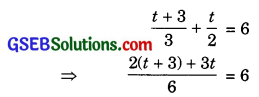⇒ 2 (t + 3) + 3t = 36
⇒ 2t + 6 + 3t = 36
⇒ 5t + 6 = 36
⇒ 5t = 36 – 6
⇒ 5t = 30
⇒ t = $$\frac{30}{5}$$ = 6
Substituting this value of t in equation (3), we get
s = 6 + 3 = 9
Therefore, the solution is
s = 9, t = 6
Verification:
Substituting s = 9 and t = 6, we find both equation (1) and (2) are satisfied as shown below:
s – t = 9 – 6 = 3
$$\frac{s}{3}+\frac{t}{2}=\frac{9}{3}+\frac{6}{2}$$
= 3 + 3 = 6
This verifies the solution.3. 3x – y = 3
9x – 3y = 9
The given pair of linear equations is
3x – y = 3 …(1)
9x – 3y = 9 …(2)
From equation (1),
y = 3x – 3 …(3)
Substituting this value of y in equation (2), we get
9x – 3(3x – 3) = 9
⇒ 9x – 9x + 9 = 0
⇒ 9 = 9
Which is true. Therefore, equations (1) and (2) have infinitely many solutions.

4. 0.2x + 0.3y = 1.3
0.4x + 0.5y = 2.3
The given system of linear equation is
0.2x + 0.3y = 1.3 …(1)
0.4x + 0.5y = 2.3 …(2)
From equation (1),
0.3y = 1.3 – 0.2x
⇒ y = $$\frac{1.3-0.2 x}{0.3}$$ …(3)
Substituting this value of y in equation (2), we get
0.4x + 0.5$$\left(\frac{1.3-0.2 x}{0.3}\right)$$ = 2.3⇒ 0.12x + 0.65 – 0.1x = 0.69
⇒ 0.12x – 0.1x = 0.69 – 0.65
⇒ 0.02x = 0.04
⇒ x = $$\frac{0.04}{0.02}$$ = 2
Substituting this value of x in equation (3), we get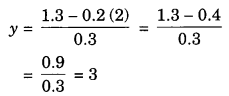Therefore, the solution is x = 2, y = 3.
Verification:
Substituting x = 2 and y = 3, we find that both the equations (1) and (2) are satisfied as shown below:
0.2x + 0.3y = (0.2) (2) + (0.3) (3)
= 0.4 + 0.9= 1.3
0.4x + 0.5y = (0.4) (2) + (0.5) (3)
= 0.8 + 1.5 = 2.23
This verifies the solution.5. $$\sqrt{2} x+\sqrt{3} y=0$$
$$\sqrt{3} x-\sqrt{8} y=0$$
The given pair of linear equations is
$$\sqrt{2} x+\sqrt{3} y=0$$ …..(1)
$$\sqrt{3} x-\sqrt{8} y=0$$ …..(2)
From equation (2),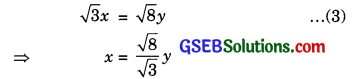Substituting this value of* in equation (1), we get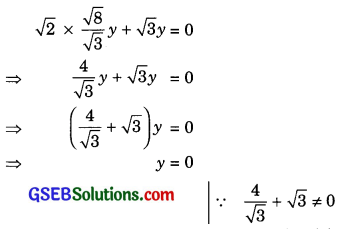Substituting this value of y in equation (3), we get
x = $$\frac{\sqrt{8}}{\sqrt{3}}(0)$$ = 0
Therefore, the solution is
x = 0, y = 0.
Verification:
Substituting x = 0 and y = 0, we find that both the equations (1) and (2) are satisfied as shown below:This verifies the solution.6. $$\frac{3 x}{2}-\frac{5 y}{3}=-2$$
$$\frac{x}{3}+\frac{y}{2}=\frac{13}{6}$$
The given system of linear equation isSubstituting the value of x in equation (4), we get
2$$\left(\frac{10 y-12}{9}\right)$$ + 3y = 13
20y – 24 + 27y = 117
47y = 117 + 24
y = $$\frac{141}{47}$$
y = 3
Substituting the value of y in equation (4), we get
2x + 3 × 3 = 13
2x + 9 = 13
2x = 13 – 9
x = $$\frac{4}{2}$$ = 2
Therefore, the solution is x = 2, y = 3
Verification:
Substituting x = 2 and y = 3, we find that both the equation (1) and (2) are satisfied as shown below: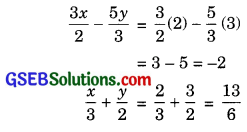This verifies the solution.Question 2.
Solve 2x + 3y = 11 and 2x – 4y = -24 and hence find the value of ‘m’ for which y = mx + 3.
Solution:
The given pair of linear equations is
2x + 3y = 11 …….(1)
2x – 4y = -24 …….(2)
From equation (1),
3y = 11 – 2x
⇒ y = $$\frac{11-2 x}{3}$$ …….(3)
Substituting this value of y in equation (2), we get
2x – 4$$\left(\frac{11-2 x}{3}\right)$$ = -24
⇒ 6x – 44 + 8x = -72
⇒ 14x – 44 = -72
⇒ 14x = 44 – 72
⇒ 14x = -28
⇒ x = $$-\frac{28}{14}$$ = -2
Substituting this value of x in equation (3), we getVerification:
Substituting x = -2 and y = 5, we find that both the equations (1) and (2) are satisfied as shown below:
2x + 3y = 2(-2) + 3(5)
= -4 + 15 = 11
2x – 4y = 2(-2) – 4(5)
= -4 – 20 = -24
This verifies the solution.
Now, y = mx + 3
⇒ 5 = m(-2) + 3
⇒ -2m = 5 – 3
⇒ -2m = 2
⇒ m = $$\frac{2}{-2}$$ = -1Question 3.
Form the pair of linear equations in the following problems and find their solution by substitution method:

1. The difference between two numbers is 26 and one number is three times the other. Find them.
2. The larger of two supplementary angles exceeds the smaller by 18 degrees. Find them.
3. The coach of a cricket team buys 7 bats and 6 balls for ₹ 3800. Later, she buys 3 bats and 5 balls for ₹ 1750. Find the cost of each bat and each ball.
4. The taxi charges in a city consist of a fixed charge together with the charge for the distance covered. For a distance of 10 km, the charge paid is ₹ 105 and for a journey of 15 km, the charge paid is ₹ 155. What are the fixed charges and the charge per kilometre? How much does a person have to pay for travelling a distance of 25 km?
5. A fraction becomes $$\frac{9}{11}$$, if 2 is added to both the numerator and the denominator. If 3 is added to both the numerator and the denominator it becomes $$\frac{5}{6}$$, find the fraction. [CBSE]
6. Five years hence, the age of Jacob will be three times that of his son, five years ago, Jacob’s age was seven times that of his son. What are their present age?

Solution:
1. Let the two numbers be x and y(x > y), then according to the question, the pair of linear equations formed is
x – y = 26 …(1)
x = 3y …(2)
Substituting the value of x from equation (2) in equation (1), we get
3y – y = 26
⇒ 2y = 26
⇒ y = $$\frac{26}{2}$$
⇒ y = 13
Substituting this value ofy in equation (2), we get
x = 3(13) = 39Hence, the required number are 39 and 13.
Verification:
Substituting x – 39 and y = 13, we find that both the equations (1) and (2) are satisfied as shown below:
x – y = 39 – 13 = 26
3y = 3(13) = 39 = x
This verifies the solution.

2. Let the larger and the smaller of two supplementary angles be x° and y° respectively.
Then, according to the question.
The pair of linear equations formed is
x° = y° + 18° …(1)
x° + y° = 180° …(2)
| ∵ The two angles are supplementary
Substituting the value of x° from equation (1) in equation (2), we get
y° + 18°+ y° = 180°
⇒ 2y° + 18° = 180°
⇒ 2y° = 180° – 18°
⇒ 2y° = 162°
⇒ y° = $$\frac{162°}{2}$$ = 81°
Substituting this value ofy° in equation (1), we get
x° = 81° + 18° = 99°Hence, the larger and the smaller of the two supplementary angles are 99° and 81° respectively.
Verification:
Substituting x° = 99° and y° = 81°, we find that both the equations (1) and (2) are satisfied as shown below:
y° + 18° = 81°+ 18° = 99° = x°
x° + y° = 99°+ 81° = 180°
This verifies the solution.

3. Let the cost of each bat and each ball be ₹ x and ₹ y respectively.
Then, according to the question, the pair of linear equations formed is
7x + 6y = 3800 …(1)
3x + 5y = 1750 …(2)
From equation (2),
5y = 1750 – 3x
y = $$\frac{1750-3 x}{5}$$ …(2)
Substituting this value of y in equation (1), we get
7x + 6$$\left(\frac{1750-3 x}{5}\right)$$ = 3800
⇒ 35x + 10500 – 18x = 19000
⇒ 17x + 10500 = 19000
⇒ 17x = 19000 – 10500
⇒ 17x = 8500
⇒ x = $$\frac{8500}{17}$$ = 500
Substituting this value of x in equation (3), we get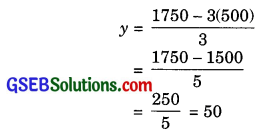Hence, the cost of each bat is ₹ 500 and each that of ball is ₹ 50 respectively.
Verification:
Substituting x = 500 and y = 50, we find that both the equations (1) and (2) are satisfied as shown below:
7x + 6y = 7(500) + 6(50)
= 3500 + 300 = 3800
3x + 5y = 3(500) + 5(50)
= 1500 + 250 = 1750
This verifies the solution.(iv) Let the fixed charges be ₹ x and the charge per kilometre be ₹ y.
Then, according to the question, the pair of
linear equations formed is
x + 10y = 105 …(1)
x + 15y = 155 …(2)
From equation (1)
x = 105 – 10y …(3)
Substituting this value of x in equation (2), we get
105 – 10y + 15y = 155
⇒ 5 + 5y = 155
⇒ 5y = 155 – 105
⇒ 5y = 50
⇒ y = $$\frac{50}{5}$$ = 10
Substituting this value of y in equation (3), we get
x = 105 – 10(10)
= 105 – 100 = 5
Hence, the fixed charges are ₹ 5 and the charge per kilometre is ₹ 10.Verification:
Substituting x = 5 and y = 10, we find that both the equations (1) and (2) are satisfied as shown below:
x + 10y = 5 + 10(10)
= 5 + 100 = 105
x + 15y = 5 + 15(10)
= 5 + 150 = 155
This verifies the solution.
Again, for travelling a distance of 25 km, a person will have to pay
= 5 + 10(25)
= 5 + 250 = ₹ 255

5. Let the fraction be $$\frac{x}{y}$$.
Then, according to the question, the pair of linear equations formed is
$$\frac{x+2}{y+2}=\frac{9}{11}$$
⇒ 11(x + 2) = 9(y + 2)
⇒ 11x + 22 = 9y + 18
⇒ 11x = 9y + 18 – 22
⇒ 11x – 9y + 4 = 0 …(1)
and $$\frac{x+3}{y+3}=\frac{5}{6}$$
⇒ 6(x + 3) = 5(y + 3)
⇒ 6x + 18 = 5y + 15
⇒ 6x – 5y = -3 …(2)From equation (1),
⇒ x = $$\frac{9 y-4}{11}$$ …(3)
Substituting this value of x in equation (2), we get
⇒ $$6\left(\frac{9 y-4}{11}\right)$$ – 5y = -3
⇒ 6(9y – 4) – 55y = -33
⇒ 54y – 24 – 55y = -33
-y + 9 = 0
y = 9
Substituting this value of y in equation (3), we getHence, the required fraction is $$\frac{7}{9}$$.
Verification:
Substituting x = 7 and y = 9, we find that both the equations (1) and (2) are satisfied as shown below: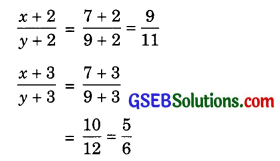This verified the solution.6. Let the present ages of Jacob and his son be x years and y years respectively.
Then, according to the question, the pair of linear equations formed is
x + 5 = 3(y + 5)
x – 5 = 7(y – 5)
⇒ x – 3y = 10 …(1)
x – 7y = -30 …(2)
From equation (1),
x = 3y + 10 …(3)
Substituting this value of x in equation (3), we get
3y + 10 – 7y = -30
-4y = -40
y = 10
Substituting y = 10 in equation (3), we get
x = 3(10) + 10
= 30 + 10 = 40
Hence, the present age of Jacob and his son are 40 years and 10 years respectively.Verification:
Substituting x = 40 and y = 10, we find that both the equations (1) and (2) are satisfied as shown below:
x – 3y = 40 – 3(10)
= 40 – 30 = 10
x – 7y = 40 – 7(10)
= 40 – 70 = -30
This verifies the solution.